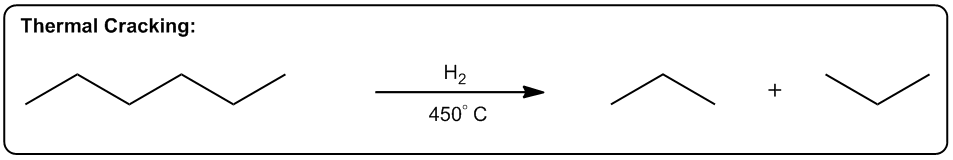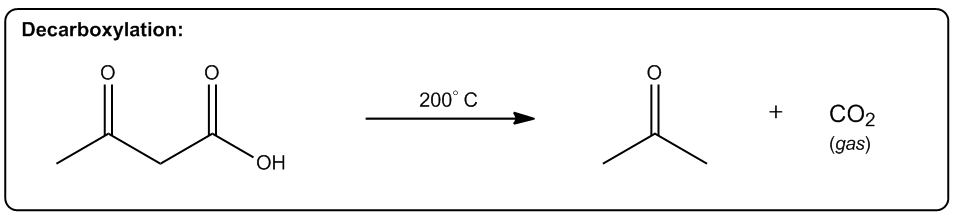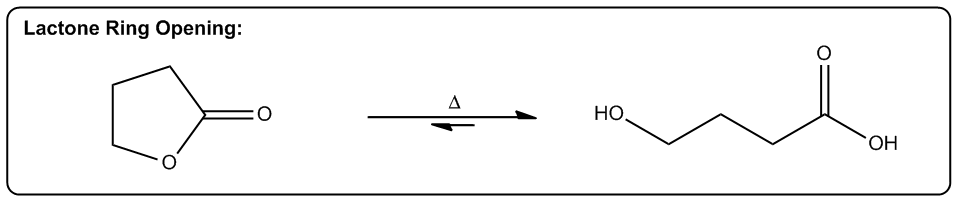Clutch Prep is now a part of Pearson
Ch. 6 - Thermodynamics and KineticsWorksheetSee all chapters

# Entropy

See all sections
Sections
Energy Diagram
Gibbs Free Energy
Enthalpy
Entropy
Hammond Postulate
Carbocation Stability
Carbocation Intermediate Rearrangements

If a reaction is exothermic, shouldn’t that be enough to determine favorability? Actually, no!

Even if a reaction is highly exothermic, the level of order it requires may make it statistically improbable.

Concept #1: Explaining what entropy is.

Transcript

All right guys, so now I want to talk about what I would consider the most confusing term of the Gibbs free energy equation and that is entropy. Entropy, generally stated, we said that it was a measure of disorder in the system. But that's a really confusing definition. I would rather go with an easier definition. What that is is that entropy is the tendency of a system to take its most probable form.
That means that if we have two different states, one is highly ordered one is not as ordered, statistically, it's more likely to be in the disordered state. That's what entropy has to do with. It has to do with probability really.
What that means is that even if a reaction is highly exothermic, like if you talk about bond association energies and enthalpy, that has to do with exothermic. But the level of order that it requires might make it statistically improbable. So basically what we do with entropy is we have to analyze is this going to be statistically more probable or statistically less probable. That is what entropy is.
Remember that we defined that entropy says that a positive, I mean, I'm sorry – a negative value is going to be more ordered because remember that basically, a positive value means that it's more disordered. That means that your entropy is getting bigger. So if you have a negative value, that means your entropy is getting smaller or it's getting more ordered.
It turns out that we're never going to have to calculate the entropy in terms of calculating the entropy of the surrounding environment or the entropy of a system because of the fact that we don't have really the tools to analyze that in orgo one. But what you are going to be asked to do is you're going to be asked to analyze if something is going to have a positive delta S or a negative delta S. That's what we want to do. We don't want to figure out the exact number. We just want to figure out is this going to be higher entropy or lower entropy.
Now there is one situation where you might calculate delta S and that's if you're given every other variable. If you're given the T and the delta H and the delta G, then sure, you could just use algebra to figure out delta S. But I'm just saying that in the absence of this being just a simple algebra problem you're not going to be asked just to calculate what the delta S is of an environment.
So let's talk about these three phenomenon that make reactions more probable or make delta S go up. And it turns out that all of them are going to be favored by high temperature. So that should be really clear in a little bit when I go back to the equation.

Entropy (ΔS) is the tendency of a system to take its most probable form.

• Negative values (-) indicate less probable = Unfavored
• Positive values (+) indicate more probable = Favored

There are 3 common ways to make reactions more probable (increase ΔS)

• They all become more likely as we add heat to the reaction (increase T)

Concept #2: 3 ways to increase entropy.

Transcript

The first one is increasing the number of molecules in a reaction, OK? So any reaction that's going to make more moles of molecules or more equivalents of molecules after the reaction is over is going to be entropically favored, why? Because what it does is it allows us to arrange these molecules in more ways, alright? So here's a really good example, thermal cracking OK? This is used by the petroleum industry to take large hydrocarbons ones that don't really do anything you can't put them in your car or anything then they put them with hydrogen in high heat, OK? And what happens is that these long hydrocarbons spontaneously start breaking into smaller pieces, OK? Why would this be a favored reaction, OK? It actually takes energy to break those bonds so why would these long hydrocarbons break? The reason is because at high enough temperature what starts to happen is that if we can break one molecule into ten molecule, right? That's going to be in entropically favored because now I can arrange those molecules in different orders, OK? So what that means is that the chances are that if I have two systems that are of equal energy one is that I have all the molecules perfectly arranged that they're all in a line or all of the molecules scattered all over the place which one is going to be more probable? The one where it's scattered, OK? And that's why thermal cracking is favored you take large hydrocarbons and you turn them into smaller ones by using high heat, high heat then makes the entropy part of the equation very very favored so now the more pieces that can break it into the more entropically favored that is, alright? This is just one example but there's lots of examples in organic chemistry of reactions that make more molecules than they start off with meaning that they're entropically favored, OK?

So let's go on to the second, the second one is a phase transition, OK? If I can transform something from a solid to a liquid or from a liquid to a gas that's going to be a more probable arrangement and the reason is because the molecules are now going to have greater vibrational freedom, OK? Remember the only difference between a solid, liquid and gas is how much energy these molecules have, OK? And how much motion they're allowed to display, in a solid there's very little vibration that can happen they can only move in a very constrained space, OK? Whereas in a gas they're bouncing all over the place for example the gas in this room right now it's bouncing off the walls it's hitting me that has a lot more freedom of motion then a solid does so if I have two systems of equal energy one where my molecules constrained to one tiny little place next to another one and then another system where the molecules can scatter as far as they want which one is more favored? The one that they can scatter in, OK? So what that means is that we have reactions that as you turn liquids into gasses or solids into liquids they're also in entropically favored, a really good example is a reaction that we're going to learn in Orgo 2 called Decarboxylation, Decarboxylation takes a Carboxylic acid at high heat, OK? And it turns it into just a ketone and CO2 gas, OK? Why is that favored? Because at high heat now the entropy part of the equation becomes more powerful it gets a higher weight, OK? And if I'm turning something into a gas that's going to be more favored because a gas is more favored than a liquid, OK? And by the way this this molecule right here would be a liquid at room temperature, OK? Now another thing to keep in mind is that this is also favored because of another thing which is this is also favored by the making more molecules rule, remember that here I have 1 molecule total at the end of the equation I have 1 molecule here and 1 molecule here so I'm going from 1 mole to 2 moles of molecules, OK? So what that means is that this is also going to be in entropically favored not just because the phase transition but also because I'm making a greater number of molecules, alright?

So let's look at the last one, the last one is increasing freedom of motion of a molecule, OK? And this specifically has to do with cyclic molecules if I can convert a cyclic molecule into in acyclic molecule that's going to be more probable because now it's going to increase the freedom of rotation of these carbon chains, OK? So this is also a reaction that you going to see a lot in Orgo 2, lactone ring opening, OK? A lactone is just a cyclic ester, OK? That's the name of a lactone we're going to talk about that a lot more in Orgo 2, what you see here is that in the presence of heat what I can do is I can take my lactone and I can break it apart into.... I can break the chain or the ring apart into two parts of the chain where this oxygen and this oxygen were linked together before and basically were the same thing, OK? Now what I do is I put one oxygen on each and now I have my carbon chain that has more freedom of motion, now why is the chain better than the ring? Because remember when we talked about if we.... Remember when we talked about conformers and we talked about single bonds being able to rotate, OK? What would happen is that these single bonds can all rotate a lot more than a ring, in a ring you actually can't rotate at all so what that means is that this one is going to have a whole lot more conformers possible than the first one, in fact the first doesn't have any, Ok? That's also more probable because if you have two of molecules of equal energy and you have one where all of the bonds are eclipsed that's what this would be all eclipsed, OK? Because I have all the Hs coming off the same side, OK? If you have one where all the Hs are eclipse and then you have another one where they're free to rotate and make anti and make gauche and make a bunch of different conformation which one is going to be more favored? The one on the side so this one is also going to be entropically favored to open that ring as they increase the heat, OK? And as I jack up the heat more that's going to favor all of these reactions and like I said the reason has to do with Gibbs free energy and remember that Gibbs free energy just basically stated that Delta G is equal to Delta H-T (delta S) so what that means is that as I increase T what I'm going to do is I'm going to increase the amount that entropy maters, OK? So if I raise all these reactions to a 1000 degrees the enthalpy isn't even going to matter anymore I'm not going to care about Bond Association energies because instead what I'm going to care about is how many molecules am I getting at the end or what's the freedom of rotation of these molecules, alright? Interesting, right? And that's going to tell us which is more statistically favored and that's going to be which one is more spontaneous, alright?

1. Increasing the Number of Molecules

Reactions that create extra molecules are more probable since there are more ways to arrange them.2. Phase Transition

Transformation of solid to liquid or liquid to gas is more probable since the molecules will have a greater vibrational freedom.3. Increasing Molecular Freedom of Motion

Converting cyclic molecules to acyclic molecules are more probable since it increases freedom of rotation.